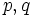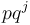# Classification of abelianness-forcing numbers

## Name

This result is attributed to Dickson, and is hence also called Dickson's theorem, though there are many results with that name.

## Statement

The following are equivalent for a natural number$n$:

1. Any group of order$n$ is an abelian group.
2.$n$ has prime factorization of the form$n = p_1^{k_1}p_2^{k_2} \dots p_r^{k_r}$ with$k_i \le 2$ for all$i$ AND$p_i$ does not divide$p_j^{k_j} - 1$ for any$1 \le i,j \le r$.

## Proof

### (1) implies (2)

It suffices to prove the contrapositive, namely, that violating the conditions of (2) allows one to construct a non-abelian group of that order. There are two subcases.

#### Case of a prime-cube dividing the number

Given: A natural number$n$ such that there exists a prime number$p$ such that$p^3$ divides$n$

To prove: There exists a non-abelian group of order$n$

Proof: First, by classification of groups of prime-cube order, there exists a non-abelian group of order$p^3$. Specifically, we can take the unitriangular matrix group:UT(3,p). Call this group$H$.

Now define:$G = H \times \mathbb{Z}/(n/p^3)\mathbb{Z}$

In other words,$G$ is the external direct product of this non-abelian group with a cyclic group of order$n/p^3$. We obtain that$G$ is non-abelian of order$n$.

#### Case of the divisibility condition being violated

Given: A natural number$n$, such that there exist distinct primes$p,q$ and a natural number$j \in \{1 , 2 \}$ such that$p$ divides$n$, and$p$ divides$q^j - 1$.

To prove: There is a non-abelian group of order$n$

Proof: Note that the product$pq^j$ divides$n$.

We can construct a non-abelian group$H$ of order$pq^j$ as follows: consider the additive group of the field of size$q^j$. The multiplicative group of this field is a cyclic group of size$q^j - 1$. Since$p$ divides$q^j - 1$, it has a subgroup of order$p$. Construct a semidirect product of the additive group of order$q^j$ with this subgroup of order$p$.

We can now construct a non-abelian group$G$ of order$n$ as the external direct product:$G = H \times \mathbb{Z}/(n/pq^j)\mathbb{Z}$

### (2) implies (1)

We prove the claim by induction on the number. Note that any divisor of a number that satisfies the condition also satisfies the condition.

Base case for induction ($n = 1$): Obvious

Inductive step: The inductive hypothesis is that the result holds for all smaller numbers.

Given: A natural number$n$ has prime factorization of the form$n = p_1^{k_1}p_2^{k_2} \dots p_r^{k_r}$ with$k_i \le 2$ for all$i$ AND$p_i$ does not divide$p_j^{k_j} - 1$ for any$1 \le i,j \le r$.$G$ is a group of order$n$.

To prove:$G$ is abelian

Proof:

Step no. Assertion/construction Facts used Give data used Previous steps used Explanation
1 Every proper subgroup of$G$ is abelian Fact (1) inductive hypothesis
arithmetic condition on$n$
Any divisor of$n$ satisfies the conditions necessary for the inductive hypothesis to apply. Applying the inductive hypothesis, we obtain that every subgroup is abelian.
2$G$ is metabelian; specifically, either$G$ is abelian or it contains an abelian maximal normal subgroup$N$ such that the quotient group$G/N$ is cyclic of prime order$p$ for some$p$ dividing$n$ Fact (2) Step (1) Step-fact combination direct
3 If$G$ is non-abelian, the maximal normal subgroup$N$ has an automorphism$\sigma$ of order$p$ Step (2) [SHOW MORE]
4$G$ must be abelian condition on no$p_i$ dividing$p_j^{k_j} - 1$ and all$k_i \le 2$ Step (3) [SHOW MORE]